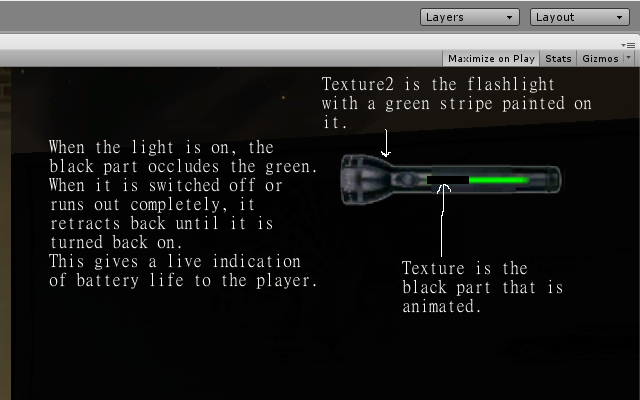# What is the best way to make a progress bar appear and reflect a change using a float?

What I’m trying to accomplish, is creating a visual horizontal indicator for a flashlight battery. I’m using an onscreen GUI element for my flashlight and want to “paint” the status bar on top of it. My script already recharges and discharges the battery dependent on a button press and the status of the light. I’d like to pick up my float, used for the amount of charge percentage, and use it to change the progress bar. I’ve been doing some reading today and there are many methods to accomplish this, but no real “best way”.

Thanks, uno.

What I’d do is multiply the width by the variable for the current battery life, divided by the max battery life.

ie.

indicatorWidth*currentBatteryLife/maxBatteryLife

Since currentBatteryLife/maxBatteryLife will give you a percent, multiplying it by the width will make set it to the same percent of the total width you’ve set.

Thanks for the response. I have already figured out what I needed to do, and your reply was part of it.

Here’s a picture of how it looks:Here’s my code:
`
var tex : Texture;
var tex2 : Texture;
var percent = 1.0;
var waveFunction : String = “sin”;
var base : float = 0.0; // start
var amplitude : float = 1.0; // amplitude of the wave
var phase : float = 0.0; // start point inside on wave cycle
var frequency : float = 0.5; // cycle frequency per second
var BatteryTimer : float; // timer for the light
var BatteryMax : float = 500;// time the light is on
var FlickerTime : float = 35;
var charging : boolean;

// Keep a copy of the original color
private var originalColor : Color;

// Store the original color
function Start ()
{
originalColor = GetComponent(Light).color;
light.enabled = false;
BatteryTimer = BatteryMax;
charging = false;
}

function OnGUI()
{
GUI.DrawTexture(Rect(1300,1,225,225), tex2);
GUI.DrawTextureWithTexCoords(Rect(1387,109,100 * (1.0 - percent),8), tex, Rect(0,0,1.0 - percent,1));
}

function Recharge()
{
if(BatteryTimer < BatteryMax)
{
BatteryTimer ++;
charging = true;
}
else
charging = false;
}

function SwitchLight()
{
var light : Light = GetComponent(Light);

`````` if(BatteryTimer >= 0 && light.enabled)
{
light.enabled = false;
Recharge();
}
else
{
light.enabled = true;
light.color = originalColor;
charging = false;
}
}
``````

function Update () {
var light : Light = GetComponent(Light);

``````  percent = BatteryTimer / BatteryMax;

if(Input.GetButtonUp("FlashLight"))
{
SwitchLight();
}

if(BatteryTimer >= 0 && light.enabled)
{
BatteryTimer --;

}
if(BatteryTimer <= FlickerTime && light.enabled)
{
light.color = originalColor * (EvalWave());
}
if(BatteryTimer <= 0 && light.enabled)
{
light.enabled = false;
Recharge();
}
if(charging)
{
Recharge();
}
}
``````

function EvalWave ()
{
var x : float = (Time.time + phase)*frequency;
var y : float;

``````  x = x - Mathf.Floor(x); // normalized value (0..1)

if (waveFunction=="sin") {
y = Mathf.Sin(x*2*Mathf.PI);
}
else if (waveFunction=="tri") {
if (x < 0.5)
y = 4.0 * x - 1.0;
else
y = -4.0 * x + 3.0;
}
else if (waveFunction=="sqr") {
if (x < 0.5)
y = 1.0;
else
y = -1.0;
}
else if (waveFunction=="saw") {
y = x;
}
else if (waveFunction=="inv") {
y = 1.0 - x;
}
else if (waveFunction=="noise") {
y = 1 - (Random.value*2);
}
else {
y = 1.0;
}
return (y*amplitude)+base;
}
``````

`
With this code you get a flashlight with a life timer, that recharges when it times out or is switched off. The cool part is the GUI interface that now displays two textures, and gives visual indication of the charge status.